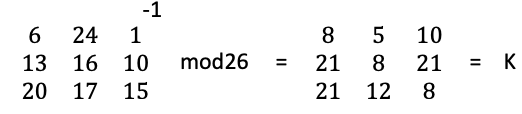# Hill Cipher

In 1929, Lester S. Hill invented the Hill Cipher, a classical cryptographic method of hiding plain text messages.

Later on, he created a cipher machine to be an aid in practicality, although it never became widespread.

The Hill Cipher is a polygraphic substitution cipher that is founded on principles from linear algebra. The cipher uses matrices, and it requires a basic understanding of such to grasp the cipher’s algorithm.

The principal mechanism of ciphering plaintext is through matrix multiplication.

Although Lester Hill is widely known for the Hill Cipher, he also contributed greatly to the design and analysis of cryptographic systems. As a mathematician, Hill was well-versed in the requisite branch for such work—number theory.

This theory is fundamental to dive deep into algorithms such as the Hill Cipher.

### Hill Cipher Example

To start, we must assign values to each character. A common scheme is a simple 0 to 25 range where A corresponds to 0 and Z corresponds to 25.

Once the scheme is set, there must be a key which will be a matrix. The key can be any matrix so long as it is a square. To simplify the example, a 3 x 3 key will be used as follows:

This key corresponds to GYB, NQK, URP when converted back to characters using the prior-defined scheme.

The following matrix is the starting point for the plaintext message “ACT”.

Now, we must multiply the plaintext by the key against mod26 to get the final ciphered message. This looks as follows:

The final matrix is then converted back to characters through the scheme to get “POH”.

Note: If you’re encrypting a message that is longer than three characters, you need to split the message up into blocks of three characters.

### Decryption

The decryption of the Hill Cipher is a relatively straightforward process. First, the encrypted message needs to be converted into a vector. Then, it needs to be multiplied by the inverse of the key.

To find the inverse, we can take K as the key matrix. We’ll also use d as the determinant of the matrix K, I as the identity matrix, and adj(K) as the matrix adjugate. While an in-depth discussion is over the top, we know the following and can K with such.

K x K-1 = I (mod26)

Then, we have:

We also know:

d x d-1 = 1(mod26)

Using the above, we can find the inverse of the original matrix as follows.Thus, the inverse of the key is IFK, VIV, VMI.

Now, multiplying the matrices of the key and the encrypted message, and using mod26, we can get back to the original plaintext.

And that is our original plaintext of “ACT”.

### Cryptanalysis of the Hill Cipher

The Hill Cipher is a complex cryptographic algorithm. However, that doesn’t mean it cannot be cracked. If you can find out the original plaintext and you also know the ciphertext, you can easily find the key.

All it takes is a set of linear equations with multiple plaintext and ciphertexts included. This is known as a known-cipher text attack.

The Hill Cipher is rarely used by itself, as it’s vulnerable to breaks. In applications where the Hill Cipher can be combined with other operations, typically non-linear, the cipher can be practical. The advantageous aspect of the Hill Cipher is the ability to achieve Shannon’s diffusion.

This is what occurs when there is only one character from the original plaintext is changed and yet the resultant ciphertext is completely unique. This is a natural phenomenon due to matrices multiplication.

### Bottom Line

The Hill Cipher is a fascinating example of more practical cryptography. While it is not a widely used cipher, it still has great utility in conjunction with others. However, the Hill Cipher also provides a good example of how complex ciphers are still subject to flaws.

In modern society, there may be many websites and applications which seem to be secure, yet they remain subject to different attacks which can expose sensitive information. If you’re concerned about your online safety, learn more about VPNs and web safety from Privacy Canada.